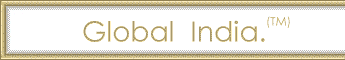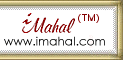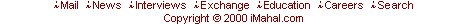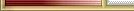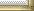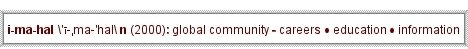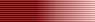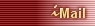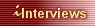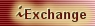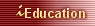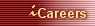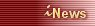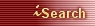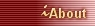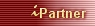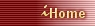Dear Mentor:

 What is the equivalent letter grade for my GPA? Archives (more questions) Ask Dear Mentor: Submit a Question

If my average overall GPA [Grade Point Average] is 3.6, what is my Average Letter Grade? You know like...A+, A, A-...etc.

The Grade Point Average (GPA) is an average of your grade points on a numeric scale of 0-4, not a letter grade. Thus, assigning a letter grade to the GPA is not a practical or a particularly useful approach, although we recognize that it may be an interesting exercise.

As you know, grade points are assigned to letter grades; for example, typical assignment is as follows: A=4, B=3, C=2, D=1 and F=0. An average of grade points on all your courses is the GPA. Some schools use the +/- system with the grades and they use a modified version of assigned grade points to the grades; for example, A+ =4.3, A =4, A- =3.7, B+ =3.3, B = 3.0, B- =2.7, and so on.

Although assigning a letter grade to the GPA is not a practical exercise, you can see that a GPA of 3.6 is just under the A- letter grade.

 Read Dear Mentor: Archives (more questions) Ask Dear Mentor: Submit a Question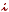ForumsRani & RajaPalsEventsAny All ExactiMahal India Directory The WebSearch Help